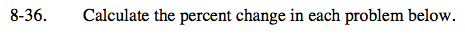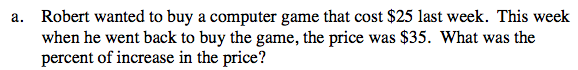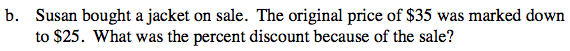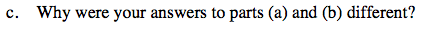Home > CC2 > Chapter 8 > Lesson 8.2.1 > Problem8-36

8-36.
1. Calculate the percent change in each problem below. Homework Help ✎

1. Robert wanted to buy a computer game that cost $25 last week. This week when he went back to buy the game, the price was$35. What was the percent of increase in the price?

2. Susan bought a jacket on sale. The original price of $35 was marked down to$25. What was the percent discount because of the sale?

3. Why were your answers to parts (a) and (b) different?Find the increase in the price and find its percentage of the original price.

Increase: $35 −$25 = $10$10 = (x)(\$25)Follow the same strategy to find the percent discount. Remember, the percent discount is with respect to the original price.

28.6%The percent increase is the amount the price increased as a percentage of the original cost.

Each percentage is based on different original prices.